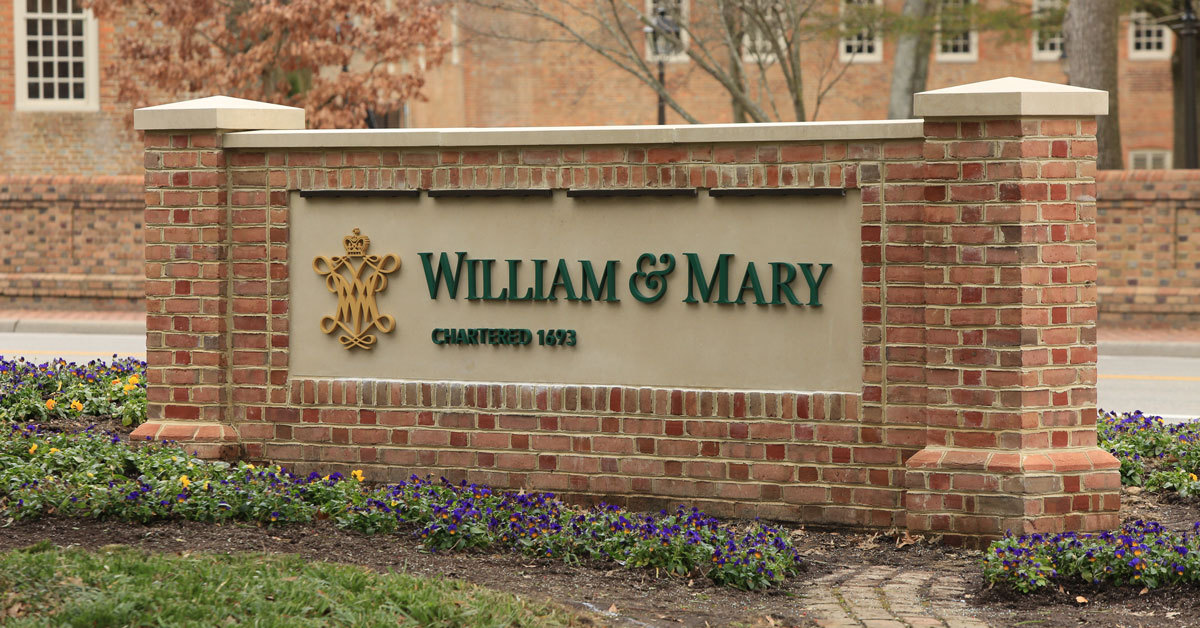Latest about COVID-19 and W&M's Path Forward.# COR Electives

These are courses that count towards the 32 credit requirement.

The following couses count towards the 32 credits required for the MS degree. They do not count towards the required seven xx8 courses. Other graduate courses may count towards the 32 credits required for the degree but must be approved by  [[rrkinc, Rex Kincaid]].

Math 551. Probability. Fall and Spring  Prerequisite(s): Consent of instructor.
Topics include: combinational analysis, discrete and continuous probability distributions and characteristics of distributions, sampling distributions.

Math 524. Operations Research II---Stochastic Models. Spring . Pre-requisite: Math 551 or equivalent probability background. A survey of probabilistic Operations Research models and applications. Topics include stochastic processes, Markov chains, queueing theory and applications, Markovian decision processes, inventory theory, and decision analysis.

Math 552. Mathematical Statistics. Fall and Spring  Prerequisite(s): Math 551 or the equivalent. The mathematical theory of statistical inference. Possible topics include: maximum likelihood, least squares, linear models, methods for estimation and hypothesis testing.

CSci 526. Simulation. Fall . Pre-requisites: Calculus, Data Structures. Introduction to simulation. Discrete and continuous stochastic models, random number generation, elementary statistics, simulation of queuing and inventory systems, Monte Carlo simulation, point and interval parameter estimation.

CSci 616. Stochastic Models in Computer Science. Fall . Pre-requisites: Knowledge of Discrete Mathematics and Calculus. An introduction to stochastic models, problem solving, and expected value analysis as applied to algorithms and systems in computer science. Topics include probability, discrete and continuous random variables, discrete-time Markov chains, and continuous-time birth-death processes.

CSci 653. Analysis of Algorithms. Fall . Pre-requisite: CSci 503 or CSci 539. Algorithm design techniques including divide-and-conquer, dynamic programming and greedy method. Analysis methods including worst case and average case. Additional topics chosen from among amortized analysis, lower bound theory and NP-completeness.

CSci 626. Data Analysis and Simulation. Fall or Spring  Staff. Pre-requisite: Knowledge of probability and statistics. Methods of discrete event simulation. Markov chains. Simulation of open and closed networks of queues. Simulation of non-stationary Poisson processes. Transient and steady-state analysis. Event list algorithms and data structures. Theoretical and empirical tests of randomness.

CSci 680. Statistical Computing. Fall . Pre-requisites: Probability, Statistics. Topics include linear regression, linear least squares, matrix factorization, nonlinear regression, Gauss-Newton methods, maximum likelihood estimation, parameter estimation, quasi-Newton methods, Monte Carlo integration, and bootstrap methods.

Pubp 616. Time Series Econometrics. Fall or Spring  Staff. Pre-requisite: Pubp 603. This course is an introduction to the econometric analysis of time series data. Topics include ARIMA models, forecasting, analysis of nonstationary series, unit root tests, co-integration and principles of modeling.